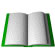# The Horn of Africa Research and Knowledge Exchange Platform :HAREPHealth Sciences Agriculture Sciences African Studies Horn of Africa Research Knowledge Org.The Horn of Africa Research and Knowledge Exchange Platform
 Science: Probability theory Acc.No Title and brief description 0001 Probability theory Category Article 0002 The evolution of probability theory Category Article 0003 Probability Theory Category educational 0004 Probability Theory Category Lecture note 0005 Probability Theory Category Article 0006 0007 Probability Theory: The Logic Of Science Category ebook 0008 Linguistic Probability Theory Category ethesis 0009 Notes on Probability Theory Category ebook 0010 Probability Theory Category Educational 0011 Introduction to Probability Category Report 0012 Statistics Tutorial: Basic Probability Category Report 0013 Probability and statistic website Category Research 0014 Probability and Statistics: Category Article

.

Pure mathematics

Mathematical Biology

Analytic physics

Discrete mathematics

Probability theory

Geometry

Algebra

Algebraic physics

Industrial Mathematicss

Computational mathematics

Mathematical linguistics

History of Mathematics

Discrete and Decision Mathematics

Mathematical Methods

Statistics